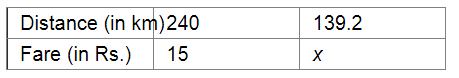# The second class railway fare for 240 km of Journey is Rs 15.00.`
Question:

The second class railway fare for 240 km of Journey is Rs 15.00. What would be the fare for a journey of 139.2 km?

Solution:

Let Rs be the fare for a journey of 139.2 km.Since the distance travelled and the fare are in direct variation, we have:

$\frac{240}{139.2}=\frac{15}{x}$

$\Rightarrow 240 \times x=15 \times 139.2$

$\Rightarrow x=\frac{15 \times 139.2}{240}$

$=\frac{2088}{240}$

$=8.7$

Thus, the fare for a journey of $139.2 \mathrm{~km}$ will be $\mathrm{Rs} 8.70$.